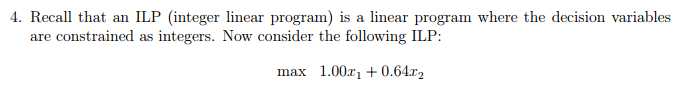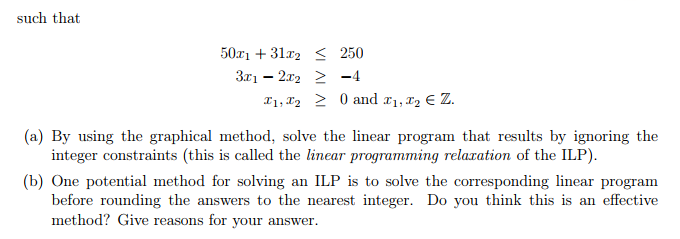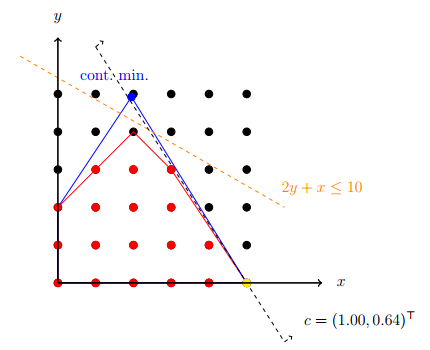# Solved: Recall that an ILP (integer linear program) is a linear program where the decision variables

(1 bookmark)Recall that an ILP (integer linear program) is a linear program where the decision variables are constrained as integers. Now consider the following ILP: max 1.00 x_1 + 0.64x_2 such that 50x_1 + 31x_2 lessthanorequalto 250 3x_1 – 2x_2 greaterthanorequalto – 4 x_1, x_2 lessthanorequalto 0 and x_1, x_2 elementof Z. (a) By using the graphical method, solve the linear program that results by ignoring the integer constraints (this is called the linear programming relaxation of the ILP). (b) One potential method for solving an ILP is to solve the corresponding linear program before rounding the answers to the nearest integer. Do you think this is an effective method? Give reasons for your answer.

In this integer linear optimization problem, the optimal solution ¯x* = (5, 0)T. If we relax the integrality restriction on x, i.e., to x ∈ R 2, we get ¯X   = (376/193, 950/193)T as the optimal solution of the LP-relaxation.

Don't use plagiarized sources. Get Your Custom Essay on
Solved: Recall that an ILP (integer linear program) is a linear program where the decision variables
GET AN ESSAY WRITTEN FOR YOU FROM AS LOW AS \$13/PAGE

Graphically, above program can be solves as:The feasible region of the LP-relaxation (blue lines), the integer feasible points (red dots) and its convex hull (red lines), the continuous minimizer x¯ = (376/193, 950/193)T (blue dot), the integer minimizer x*= (5, 0)T(yellow dot), a cutting plane (orange dashed line) and the level set {x ∈ R2 | cTx = 5} (black dashed line).

(B) Solving an integer linear program by solving a corresponding linear program before rounding the answers to the nearest integer is not an effective method because of below reasons:

• Taking a solution and rounding may give something that is no longer a solution.
• Even if the result of the rounding is a solution, there is no guarantee that it is the best out of all solutions that uses integers.
• Sometimes there are both constraints that place lower limits and constraints that place upper limits.